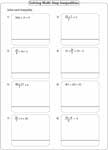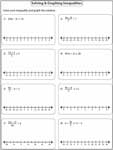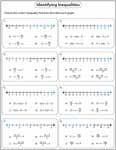Multi-Step Inequalities Worksheets

As the name says, multi-step inequality takes more than two steps to solve the problem. This page provides the best-practice resources in solving and graphing multi-step inequalities. Multiple-choice questions are given in three major forms, identifying graphs, inequalities and interval notation.

Circle the Correct Solutions

Plug in the values for each multi-step inequality and identify the correct solution.Solving Multi-Step Inequalities

Solve each multi-step inequality. There are eight problems in each worksheet. Difficult levels contain algebraic expressions in fractions.Easy:

Moderate:

Difficult:

Decimals:

Solving and Graphing Multi-Step Inequalities

Solve each multi-step inequality and graph the solution. Please use blank paper for calculations.Easy:

Moderate:

Difficult:

Decimals:

Multiple Choices: Interval Notation

Each worksheet has eight multiple-choice questions with solutions in interval notation.Easy:

Moderate:

Multiple Choices: Identifying Graphs

There are four graphs in each question. Solve the inequality and identify the correct solution graph.Easy:

Moderate:

Multiple Choices: Identifying Inequalities

Solve each inequality from the choices and identify the one that has the given solution graph.Easy:

Moderate:

Stock up your library with the complete set of worksheets on multi-step inequalities.

Related Worksheets